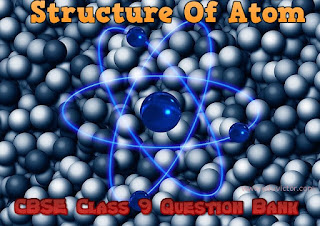## Structure Of Atom

(Question Bank)

Questions Based on Class 9 NCERT Science Chapter

Q.1: Define atomic number.

Q.2: What is an electron? Who discovered it?

Q.3: Name the particles which determine the mass of an atom.

Q.4: Study the composition of the two nuclei of two atomic species L and M
L = protons 20, neutrons 20
M = protons 18, neutrons 22
Give the relation between the two.

Q.5: Write the formula for calculating the maximum number of electrons in an orbit.

Q.6: Name the particles that have no charge and their mass is equal to that of proton. Who discovered these particles?

Q.7: What is charge/mass ratio of an electron?

Q.8: What is the valency of oxygen [Atomic number of oxygen=8]?

Q.9: Write the notation of an atom ‘X’, if the mass number is A and the atomic number is Z.

Q.10: If the electronic configuration of an atom X is 2,8,1, calculate the total number of electrons and write the name of an atom.

Q.11: What will be the number of neutrons if an atom has mas number=23 and the number of electrons=11?

Q.12: What will happen to an element ‘Z’ if its atoms gain three electrons?

Q.13: There are 15 protons and 16 neutrons in the nucleus of an element. Calculate its atomic number and its atomic mass.

Q.14: Give one word for the following:
(i) Positively charged atom.
(ii) A group of atoms carrying a charge.

Q.15: Which atom, Na+ or He, has completely filled K and L shells? Give reason to support your answer.

Q.16: The atomic number of atoms of two elements are 18 and 20 respectively and their mass number is 40. Mention the term that can be given to relate such pairs of atoms. Will they have the same chemical properties? Justify your answer.

Q.17: How many electrons, protons and neutrons will be there in an element 199X? What will be the valency of the element?

Q.18: An atom of an element has three electrons in its 3rd orbit, which is the outermost shell. Write:
(i) the electronic configuration
(ii) atomic number
(iii) number of protons
(iv) valency

Q.19: Composition of the nuclei of two atoms ‘X’ and ‘Y’ are given below:
X Y
Protons = 4 4
Neutrons = 4 6

Give the mass number of X and Y. Mention the relationship between the two atoms.

Q.20: (a) J. Chadwick discovered a sub-atomic particle that has no charge and has mass nearly equal to that of a proton. Name the particle and give its location in the atom.

(b) If ‘K’ and ‘L’ shells of an atom are completely filled, then what would be:
(i) the total number of electrons in the atom and
(ii) Its valency?

Q.21: The electronic configuration of an element ‘X’ is 2,8,2:

(a) Find the number of electrons present in the atom of element ‘X’.

(b) Write its atomic number.

(c) Is element ‘X’ a metal or a non-metal?

(d) Find out the valency of the element ‘X’.

Q.22: An element ‘Z’ forms the following compound when it reacts with hydrogen, chloride, oxygen and phosphorous.
ZH3,ZCl3,Z2O3 and ZP

(a) What is the valency of element Z?

(b) Element ‘Z’ is metal or non metal?

Q.23: Elements from A to E have in them the distribution of e,p and n as follows:

Elements Electrons Neutrons Protons
A 4 4 3
B 8 9 9
C 18 22 18
D 17 18 17

Making use of these data find
(i) cation
(ii) anion
(iii)  a pair of isotopes
(iv) an atom of noble gas

Q.24: An atom of an element has one electron in the outer most M shell. State its:
(a) Electronic configuration
(b) Number of protons
(c) Atomic number
(d) Valency of this element

Q.25: The discovery of subatomic particles led to a revolution in the study of matter. Name the scientist who discovered these sub-atomic particles.

Q.26: List the observations in α-particle scattering experiment which led Rutherford to make the following conclusions:
(i) Most of the space in an atom is empty.
(ii) Whole mass of an atom is concentrated in its centre.
(iii) Centre is positively charged.

Q.27: (a) Which isotope is used in the treatment of cancer?

(b) A particle contains 11 protons and 10 electrons. Write the symbol and the name of the particle.

(c) What do you think will be the observation if the alpha-particle scattering experiment is carried out using a foil of a metal other than gold?

Q.28: State the difference between isotope and isobar. Give an example of each.

Q.29: identify two pairs of elements that will have similar chemical properties. Write the relation between these elements.

Q.30: State three characteristic of Canal rays.

Q.31: (a) Write the electronic configuration of an element X whose atomic number is 15.

(b) An isotope of chlorine is represented as 3717Cl. Calculate the number of electrons, protons and neutrons.

(c) What are isobars?1.2.